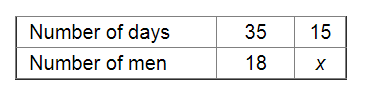# 18 men can reap a field in 35 days.`
Question:

18 men can reap a field in 35 days. For reaping the same field in 15 days, how many men are required?

Solution:

Let the number of men required to reap the field in 15 days be x.Since the number of days and the number of men required ro reap the field are in inverse variation, we have:

$35 \times 18=15 \times x$

$\Rightarrow x=\frac{35 \times 18}{15}$

$=42$

Thus, the required number of men is 42 .